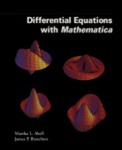## Mathematical Sciences Faculty Bookshelf#### Title

Differential Equations with Mathematica

#### Files

Download Full Text

#### Description

Georgia Southern faculty members Martha L. Abell and James P. Braselton co-authored Differential Equations with Mathematica.

Differential Equations with Mathematica presents an introduction and discussion of topics typically covered in an undergraduate course in ordinary differential equations as well as some supplementary topics such as Laplace transforms, Fourier series, and partial differential equations. It also illustrates how Mathematica is used to enhance the study of differential equations not only by eliminating the computational difficulties, but also by overcoming the visual limitations associated with the solutions of differential equations.

The book contains chapters that present differential equations and illustrate how Mathematica can be used to solve some typical problems. The text covers topics on differential equations such as first-order ordinary differential equations, higher order differential equations, power series solutions of ordinary differential equations, the Laplace Transform, systems of ordinary differential equations, and Fourier Series and applications to partial differential equations. Applications of these topics are provided as well.

Engineers, computer scientists, physical scientists, mathematicians, business professionals, and students will find the book useful.

10-18-1993

Academic Press

#### ISBN for this edition (13-digit)

9781483213910COinS